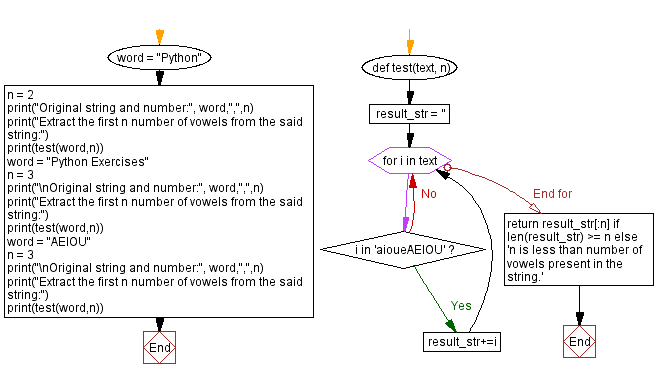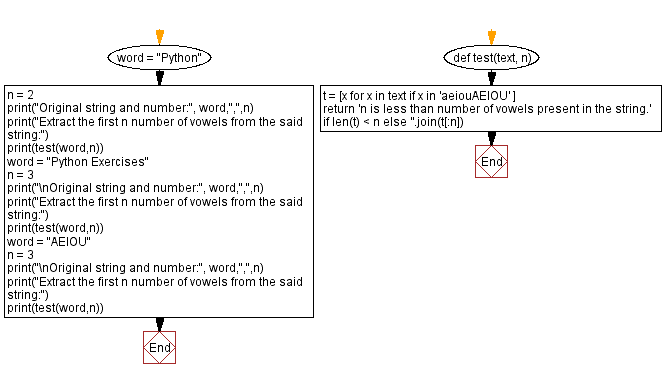﻿ Python - Extract the first n number of vowels from a string# Python Exercises: Extract the first n number of vowels from a string

## Python List: Exercise - 280 with Solution

A vowel is a syllabic speech sound pronounced without any stricture in the vocal tract. Vowels are one of the two principal classes of speech sounds, the other being the consonant.

Write a Python program to extract the first specified number of vowels from a given string. If the specified number is less than number of vowels present in the string then display “n is less than number of vowels present in the string”.

Sample Data:
("Python", 2) -> "n is less than number of vowels present in the string."
("Python Exercises", 3) -> "oEe"
("aeiou") -> "AEI"

Sample Solution-1:

Python Code:

``````def test(text, n):
result_str = ''
for i in text:
if i in 'aioueAEIOU':
result_str+=i
return result_str[:n] if len(result_str) >= n else 'n is less than number of vowels present in the string.'

word = "Python"
n = 2
print("Original string and number:", word,",",n)
print("Extract the first n number of vowels from the said string:")
print(test(word,n))
word = "Python Exercises"
n = 3
print("\nOriginal string and number:", word,",",n)
print("Extract the first n number of vowels from the said string:")
print(test(word,n))
word = "AEIOU"
n = 3
print("\nOriginal string and number:", word,",",n)
print("Extract the first n number of vowels from the said string:")
print(test(word,n))
```
```

Sample Output:

```Original string and number: Python , 2
Extract the first n number of vowels from the said string:
n is less than number of vowels present in the string.

Original string and number: Python Exercises , 3
Extract the first n number of vowels from the said string:
oEe

Original string and number: AEIOU , 3
Extract the first n number of vowels from the said string:
AEI
```

Flowchart:## Visualize Python code execution:

The following tool visualize what the computer is doing step-by-step as it executes the said program:

Sample Solution-2:

Python Code:

``````def test(text, n):
t = [x for x in text if x in 'aeiouAEIOU' ]
return 'n is less than number of vowels present in the string.' if len(t) < n else ''.join(t[:n])

word = "Python"
n = 2
print("Original string and number:", word,",",n)
print("Extract the first n number of vowels from the said string:")
print(test(word,n))
word = "Python Exercises"
n = 3
print("\nOriginal string and number:", word,",",n)
print("Extract the first n number of vowels from the said string:")
print(test(word,n))

word = "AEIOU"
n = 3
print("\nOriginal string and number:", word,",",n)
print("Extract the first n number of vowels from the said string:")
print(test(word,n))
```
```

Sample Output:

```Original string and number: Python , 2
Extract the first n number of vowels from the said string:
n is less than number of vowels present in the string.

Original string and number: Python Exercises , 3
Extract the first n number of vowels from the said string:
oEe

Original string and number: AEIOU , 3
Extract the first n number of vowels from the said string:
AEI
```

Flowchart:## Visualize Python code execution:

The following tool visualize what the computer is doing step-by-step as it executes the said program:

Python Code Editor:

Have another way to solve this solution? Contribute your code (and comments) through Disqus.

Previous Python Exercise: Check if each number is prime in a list of numbers.
Next Python Exercise: Find all pairs of that differ by three in a list.

What is the difficulty level of this exercise?

Test your Programming skills with w3resource's quiz.

﻿

## Python: Tips of the Day

Getting sorted iterable (can sort by "compare" function):

```>>> a = [1, 2, -3]
>>> sorted(a)
[-3, 1, 2]

>>> sorted(a,key=abs)
[1, 2, -3]
```